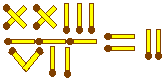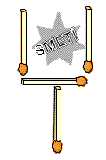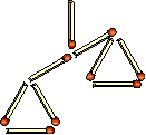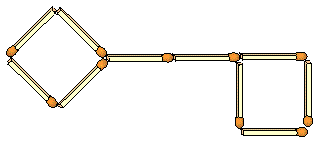LANGUAGE:

# Matchstick Puzzles

## Matchstick Equations

Try to rectify a mistake by moving a single matchstick, to get the correct equation.

The following equation is made of 11 matches:
XI - V = IV (more solutions)

The following equation is made of 11 matches:
X + V = IV (more solutions)

The following equation is made of 10 matches:
L + L = L (more solutions)

The following equation is made of 12 matches:
VI = IV - III (more solutions)

The following equation is made of 14 matches:
XIV - V = XX

The following equation is made of 11 matches:
IX - IX = V

The following equation is made of 12 matches:
X = VIII - II

The following equation is made of 7 matches:
VII = I

## Tricky Matchstick Equation

Move one matchstick to get the correct equation.## 4 Identical Triangles

Move one matchstick to get 4 identical triangles.## Shovel

Move just two matches and remove dust from the shovel.## House

1. Move just two matches to make eleven squares.
2. Move four matches and form 15 squares.## Scales

Move 5 matches to make the scales balanced.## Fish

Move just 3 matches so that the fish swims the other direction.## Rabbit Hutch

In the picture there are little flats for 6 rabbits. Can you build a dwelling for these 6 rabbits with only 12 matches? 6 separate flats are needed for the rabbits.## Cow

This cow has the following parts: head, body, horns, legs and tail. It is looking to the left. Move two matches so that it is looking to the right.## Key

1. Move four matches so that three squares are created.
2. Move three matches so that two rectangles are created.
3. Move two matches so that two rectangles are created.## Touch

Place six matches in such a way that each match is in touch with all the other five matches.